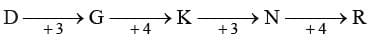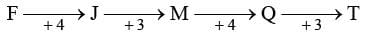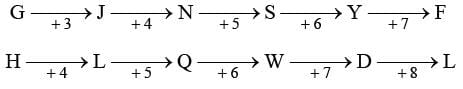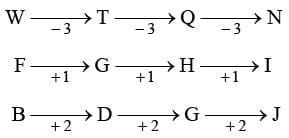# Olympiad Test: Alphabet Test - 1

## 10 Questions MCQ Test Mathematical Olympiad Class 8 | Olympiad Test: Alphabet Test - 1

Description
Attempt Olympiad Test: Alphabet Test - 1 | 10 questions in 20 minutes | Mock test for Class 8 preparation | Free important questions MCQ to study Mathematical Olympiad Class 8 for Class 8 Exam | Download free PDF with solutions
QUESTION: 1

### If ZOO stands for 56, DEER stands for 32 then for which numerical value LION stands for?

Solution:

Z = 26; O = 15.
ZOO = 26 + 15 + 15 = 56.
D = 4, E = 5; R = 18.
DEER = 4 + 5 + 5 + 18 = 32.
LION = 12 + 9 + 15 + 14 = 50

QUESTION: 2

### If JEANS = 49, COAT = 39 then SHIRT = ?

Solution:

JEANS = 10 + 5 + 1 + 14 + 19 = 49.
COAT = 3 + 15 + 1 + 20 = 39.
SHIRT = 19 + 8 + 9 + 18 + 20 = 74

QUESTION: 3

### If BUD = 27, ROSE = 57 then FLOWER = ?

Solution:

BUD = 2 + 21 + 4 = 27
ROSE = 18 + 15 + 19 + 5 = 57
FLOWER = 6 + 12 + 15 + 23 + 5 + 18 = 79

QUESTION: 4

BMX, DNW, FOU, ?

Solution:

BMX, DNW, FOU, HPT

QUESTION: 5

UPI, SHJ, ODP, MBQ, ?

Solution:

UPI, SHJ, ODP, MBQ, IAW.

QUESTION: 6

AYD, BVF, ? , GMJ.

Solution:

AYD, BVF, DSH, GMJ.

QUESTION: 7

BMZ, EPY, HSX, ?

Solution:

BMZ, EPY, HSX, KVW.

QUESTION: 8

DF, GJ, KM, NQ,?

Solution:QUESTION: 9

GH, JL NQ, SW, YD, ?

Solution:QUESTION: 10

WFB, TGD, QHG, ?

Solution:Use Code STAYHOME200 and get INR 200 additional OFF Use Coupon Code